## Be a geoscience and data science detective

### Featured

September 16, 2020

#### Introduction

These days everyone talks about data science. But here’s a question: if you are a geoscientist, and like me you have some interest in data science (that is, doing more quantitative and statistical analyses with data), why choose between the two? Do both… always! Your domain knowledge is an indispensable condition, and so is an attitude of active curiosity (for me, an even more important condition). So, my advice for aspiring geoscientists and data scientists is: “yes, do some course work, read some articles, maybe a book, get the basics of Python or R, if you do not know a programming language” but then jump right ahead into doing! But – please! – skip the Titanic, Iris, Cars datasets, or any other data from your MOOC or that many have already looked at! Get some data that is interesting to you as geoscientist, or put it together yourself.

#### First example: evaluate the quality of seismic inversion from a published article

In this first example, the one presented at Transform 2020, I wanted to evaluate the quality of seismic inversion from a published article in the November 2009 CSEG Recorder Inversion Driven Processing. In the article, the evaluation was done by the authors at a blind well location, but only qualitatively, as illustrated in Figure 5, shown below for reference. In the top panel (a) the evaluation is for the inversion without additional processing (SPNA, Signal Protected Noise Attenuation); in the bottom panel (b) the evaluation is for the inversion with SPNA. On the right side of each panel the inverted seismic trace is plotted against the upscaled impedance well log (calculated by multiplying the well density log and the well velocity log from compressional sonic); on the right, the upscaled impedance log is inserted in a seismic impedance section as a colored trace (at the location of the well) using the same color scale and range used for the impedance section.Figure 5 caption: Acoustic impedance results at the blind well for data without (a) and with (b) SPNA. The figure shows a 200 ms window of inverted seismic data with well B, the blind well, in the middle on the left, along with acoustic impedance curves for the well (red) and inverted seismic (blue) on the right. The data with SPNA shows a better fit to the well, particularly over the low frequencies.

What the authors reported in the figure caption is the extent to which the evaluation was discussed in the paper; unfortunately it is not backed up in any quantitative way, for example comparing a score, such as R^2, for the two methods. Please notice that I am not picking on this paper in particular, which in fact I rather quite liked, but I am critical of the lack of supporting statistics, and wanted to supplement the paper with my own.

In order to do that, I hand-digitized from the figure above the logs and inversion traces , then interpolated to regularly-sampled time intervals (by the way: if you are interested in a free tool to  digitize plots, check  use WebPlotDigitizer).

My plan was to split my evaluation in an upper and lower zone, but rather than using the seismically-picked horizon, I decided to add a fake top at 1.715 seconds, where I see a sharp increase in impedance in Figure 5. This was an arbitrary choice on my part of a more geological horizon, separating the yellow-red band from the green blue band in the impedance sections. The figure below shows all the data in a Matplotlib figure:The first thing I did then, was to look at the Root Mean Square Error in the upper and lower zone obtained using the fake top. They are summarized in the table below: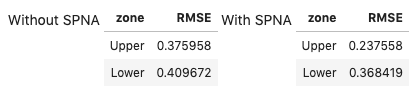Based on the RMSE , it looks like case b, the inversion with Signal Protected Noise Attenuated applied on the data, is a better result for the `Upper` zone, but not for the `Lower` one. This result is in agreement with my visual comparison of the two methods.

But lets’ dig a bit deeper. After looking at RMSE, I used the updated version of the `error_flag` function, which I first wrote at the 2018 Geophysics Sprint, listed below:

```def error_flag(pred, actual, stat, dev = 1.0, method = 1):
"""Calculate the difference between a predicted and an actual curve
and return a curve flagging large differences based on a user-defined distance
(in deviation units) from either the mean difference or the median difference

Matteo Niccoli, October 2018. Updated in May 2020.

Parameters:
predicted : array
predicted log array
actual : array
original log array
stat : {‘mean’, ‘median’}
The statistics to use. The following options are available:
- mean: uses numpy.mean for the statistic,
and np.std for dev calculation
- median: uses numpy.median for the statistic,
and scipy.stats.median_absolute_deviation (MAD) for dev calculation
dev : float, optional
the standard deviations to use. The default is 1.0
method : int {1, 2, 3}, optional
The error method to use. The following options are available
(default is 1):
1: difference between curves larger than mean difference plus dev
2: curve slopes have opposite sign (after a 3-sample window smoothing)
3: curve slopes of opposite sign OR difference larger than mean plus dev
Returns:
flag : array
The error flag array
"""

flag = np.zeros(len(pred))
err = np.abs(pred-actual)

if stat == 'mean':
err_stat = np.mean(err)
err_dev = np.std(err)
elif stat == 'median':
err_stat = np.median(err)
err_dev = sp.stats.median_absolute_deviation(err)

pred_sm = pd.Series(np.convolve(pred, np.ones(3), 'same'))
actual_sm = pd.Series(np.convolve(actual, np.ones(3), 'same'))
ss = np.sign(pred_sm.diff().fillna(pred_sm))
ls = np.sign(actual_sm.diff().fillna(actual_sm))

if method == 1:
flag[np.where(err>(err_stat + (dev*err_dev)))] = 1
elif method == 2:
flag[np.where((ss + ls)==0 )]= 1
elif method == 3:
flag[np.where(np.logical_or(err>(err_stat + (dev*err_dev)), (ss+ls)==0 ))]= 1
return flag```

I believe this new version is greatly improved because:

• Users now can choose between mean/standard deviation and median/median absolute deviation as a statistic for the error. The latter is more robust in the presence of outliers
• I added a convolutional smoother prior to the slope calculation, so as to make it less sensitive to noisy samples
• I expanded and improved the doctstring

The figure below uses the flag returned by the function to highlight areas of poorer inversion results, which I assigned based on passed to the function very restrictive parameters:

• using median and a median absolute deviation of 0.5 to trigger flag
• combining the above with checking for the slope signI also wrote short routines to count the number and percentage of samples that have been flagged, for each result, which are summarized in the table below: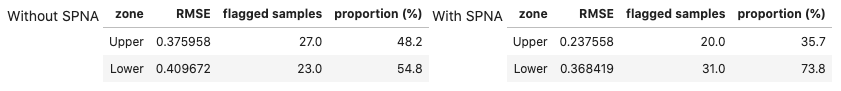The error flag method is in agreement with the RMS result: case b, the inversion on Signal Protected Noise Attenuated data is a better result for the `Upper` zone, but for the `Lower` zone the inversion without SPNA is the better one. Very cool!

But I was not satisfied yet. I was inspired to probe even deeper after a number of conversations with my friend Thomas Speidel, and reading the chapter on Estimation from Computational and Inferential Thinking (UC Berkeley). Specifically, I was left with the question in mind: “Can we be confident about those two is better in the same way?

This question can be answered with a bootstrapped Confidence Interval for the proportions of flagged samples, which I do below using the code in the book, with some modifications and some other tools from the datascience library. The results are shown below. The two plots, one for the `Upper` and one for the `Lower` zone, show the distribution of bootstrap flagged proportions for the two inversion results, with SPNA in yellow, and without SPNA in blue, respectively, and the Confidence Intervals in cyan and brown, respectively (the CI upper and lower bounds are also added to the table, for convenience).

By comparing the amount (or paucity) of overlap between the distributions (and between the confidence intervals) in the two plots, I believe I can be more confident in the conclusion drawn for the Lower zone, which is that the inversion on data without SPNA is better (less proportion of flagged errors), as there is far less overlap.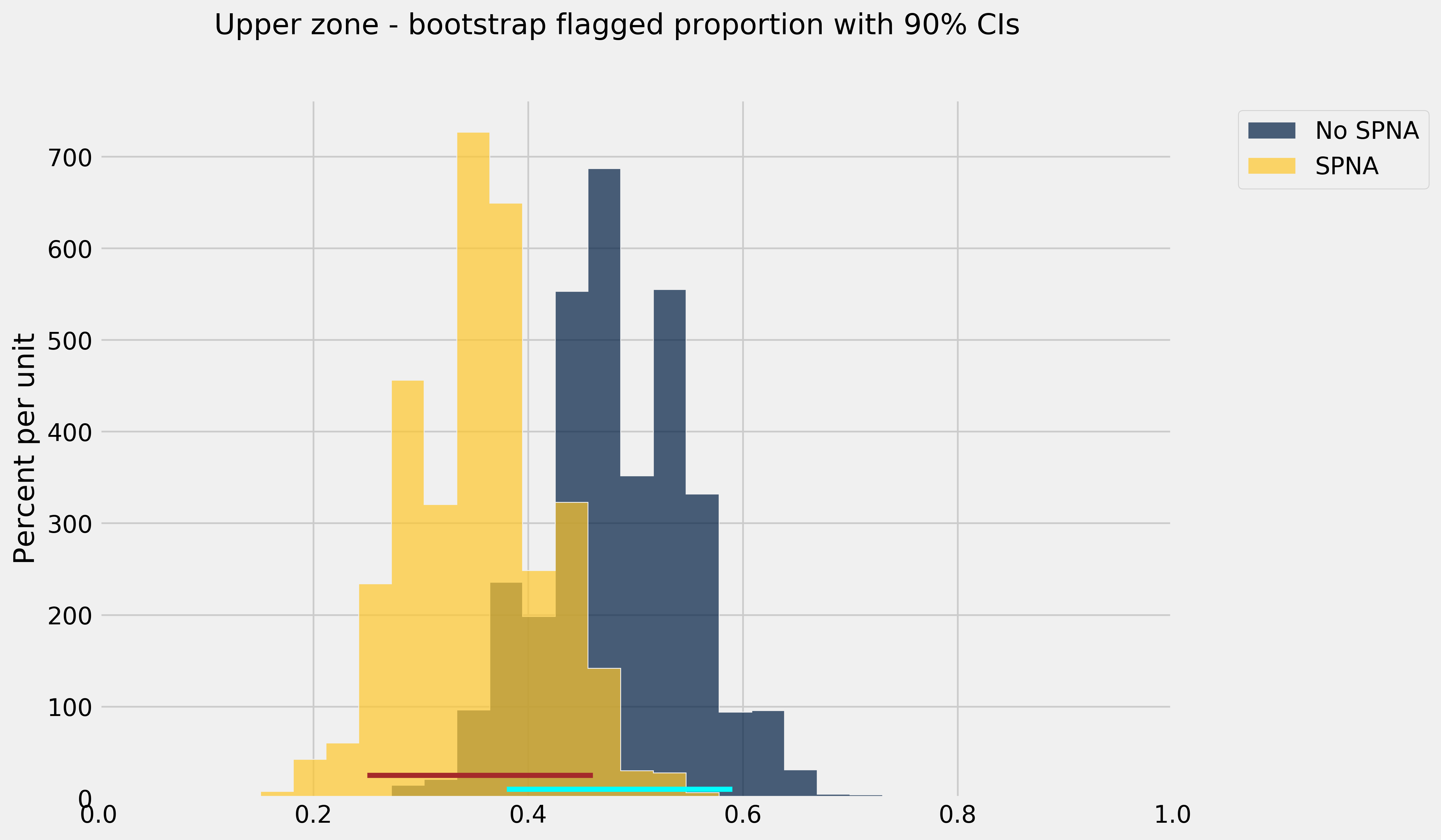I am very pleased with these results. Of course, there are some caveats to keep in mind, mainly that:
• I may have introduced small, but perhaps significant errors with hand digitizing
• I chose a statistical measure (the median and median absolute deviation) over a number of possible ones
• I chose an arbitrary geologic reference horizon without a better understanding of the reservoir and the data, just the knowledge from the figure in the paper

However, I am satisfied with this work, because it points to a methodology that I can use in the future. And I am happy to share it! The Jupyter notebook is available on GitHub.

#### Second example: evaluate regression results from a published article

My second, more recent example, is shorter but no less interesting, in my opinion. It is a case study correlating inversion data to petrophysical estimates of porosity-height in the Doig and Montney Formations in Western Canada (from the paper Tight gas geophysics: AVO inversion for reservoir characterization, Close et al. CSEG Recorder, May 2010, an article which I enjoyed very much reading).

The authors indicated that Vp/Vs and/or Poisson’s ratio maps from seismic inversion are good indicators of porosity in the Lower Doig and Upper Montney reservoirs in the wells used in their study, so it was reasonable to try to predict Phi-H from Vp/Vs via regression. The figure below, from the article, shows one such Vp/Vs ratio map and the Vp/Vs vs. Phi-H cross-plot for 8 wells.Figure 7 caption: Figure 7. a) Map of median Vp/Vs ratio value and porosity-height from 8 wells through the Lower Doig and Upper Montney. The red arrows highlight wells with very small porosity-height values and correspond in general to areas of higher Vp/Vs ratio. The blue arrow highlights a well at the edge of the seismic data where the inversion is adversely affected by decreased fold. The yellow line is the approximate location of a horizontal well where micro-seismic data were recorded during stimulation. b) Cross-plot of porosity-height values as shown in (a) against Vp/Vs extracted from the map. The correlation co-efficient of all data points (blue line) of 0.7 is improved to 0.9 (red line) by removing the data point marked by the blue arrow which again corresponds to the well near the edge of the survey (a).

They also show in the figure that by removing one of the data points, corresponding to a well near the edge of the survey (where the seismic inversion result is presumably not as reliable, due to lower offset and azimuth coverage), the correlation co-efficient is improved from 0.7 to 0.9 (red line).

So, the first thing I set out to do was to reproduce the crossplot.  I again hand-digitized the porosity-height and Vp/Vs pairs in the cross-plot using again WebPlotDigitizer. However, switched around the axes, which seems more natural to me since the objectives of regression efforts would be to predict as the dependent variable Phi-h, at the location of yet to drill wells, given Vp/Vs from seismic inversion. I also labelled the wells using their row index, after having loaded them in a Pandas DataFrame. And I used Ordinary Least Square Regression from the `statsmodels` library twice: once with all data points, the second time after removal of the well labelled as 5 in my plot above.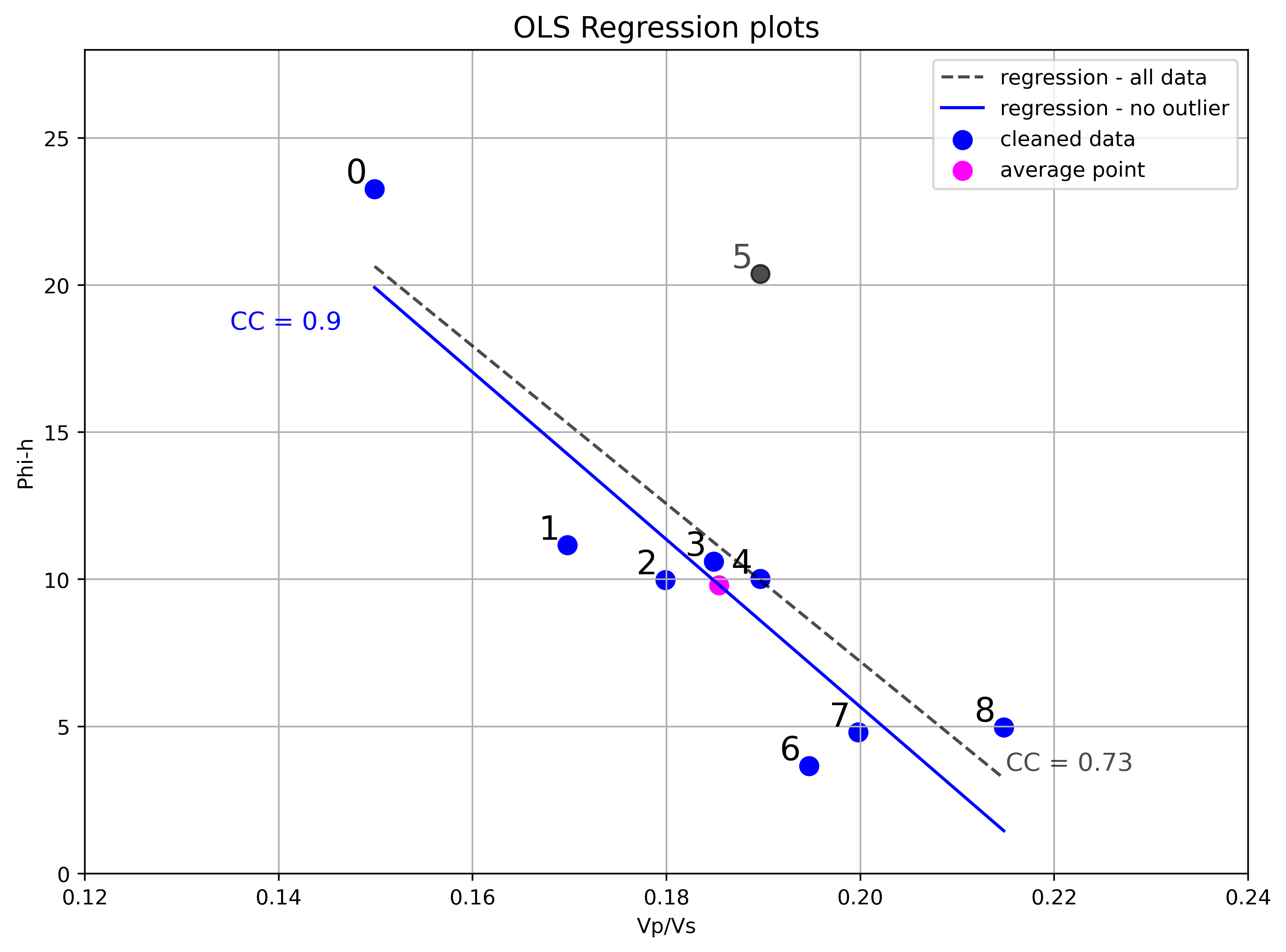So, I am able to reproduced the analysis from the figure. I think removing an outlier with insight from domain knowledge (the observation that poorer inversion result at this location is reasonable for the deviation from trend) is a legitimate choice. However, I would like to dig a bit deeper, to back up the decision with other analyses and tests, and to show how one might do it with their own data.

The first thing to look at is an Influence plot, which is a plot of the residuals, scaled by their standard deviation, against the leverage, for each observation. Influence plots are useful to distinguish between high leverage observations from outliers and are one of `statsmodel` ‘s standard Regression plots, so we get the next figure almost for free, with minor modifications to the default example). Here it is below, together with the OLS regression result, with all data points.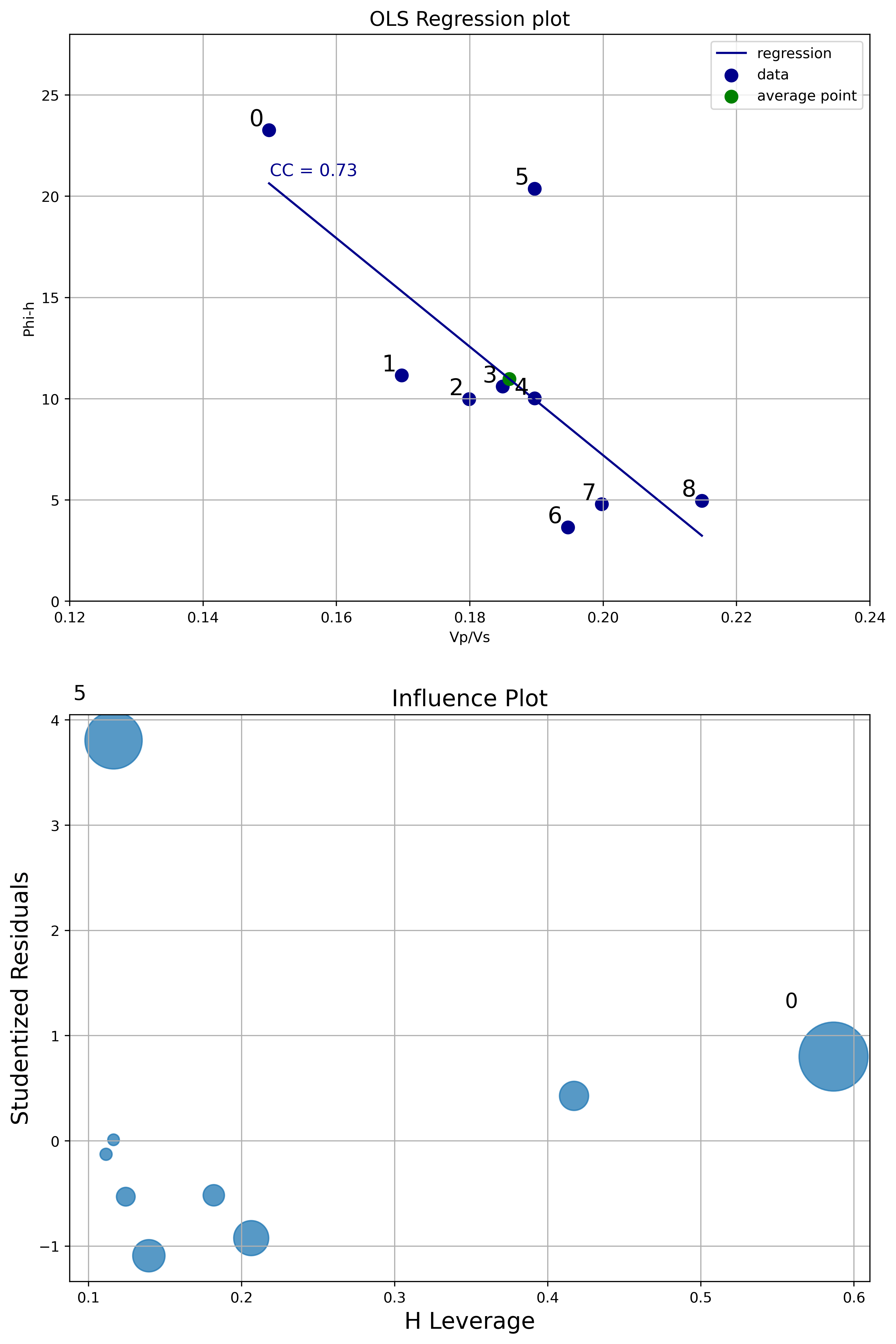From the Influence plot it is very obvious that the point labelled as zero has high leverage (but not high normalized residual). This is not a concern because points with high leverage are important but do not alter much the regression model. On the other hand, the point labelled as 5 has very high normalized residual. This point is an outlier and it will influence the regression line, reducing the R^2 and correlation coefficient. This analysis is a robust way to legitimize removing that data point.

Next I run some inferential tests. As I’ve written in an earlier notebook on Data loading, visualization, significance testing, I find the critical r very useful in the context of bivariate analysis. The critical r is the value of the correlation coefficient at which you can rule out chance as an explanation for the relationship between variables observed in the sample, and I look at it in combination with the confidence interval of the correlation coefficient.

The two plots below display, in dark blue and light blue respectively, the upper and lower confidence interval bounds, as the correlation coefficient r varies between 0 and 1 (x axis). These two bounds will change with different number of wells (they will get closer with more wells, and farther apart with less wells). The lower bound intersects the x axis (y=0) axis at a value equal to the critical r (white dot). The green dots highlight the actual confidence interval for a specific correlation coefficient chosen, in this case 0.73 with 9 wells, and 0.9 with 8 wells.

By the way: these plots are screen captures from the interactive tool I built taking advantage of the Jupyter interactive functionality (`ipywidgets`). You can try the tool by running the Jupyter notebook.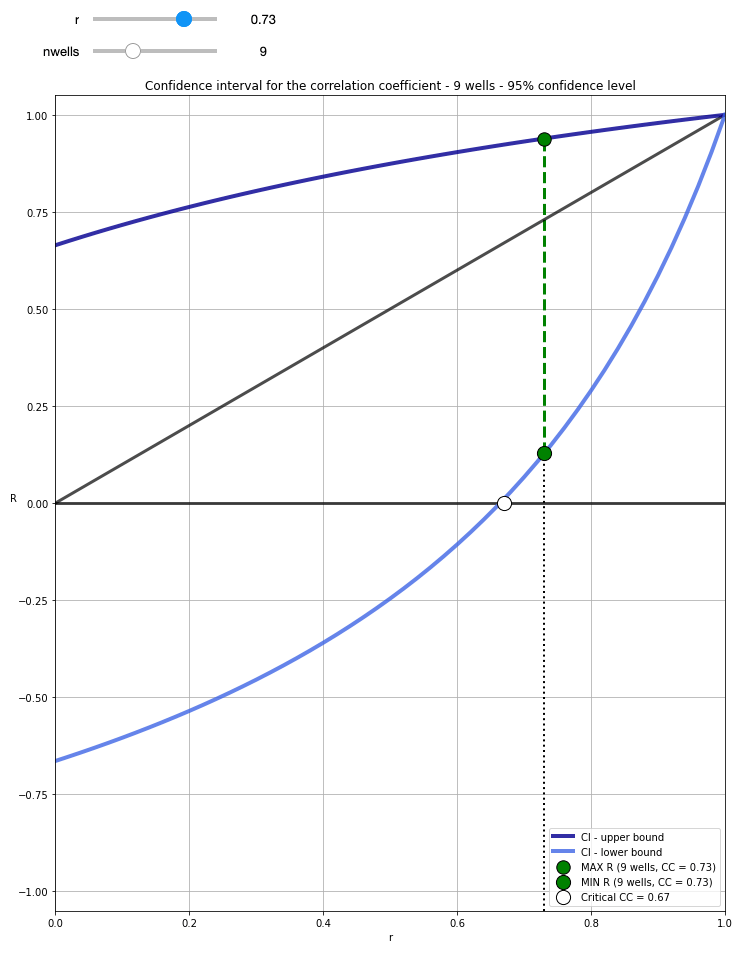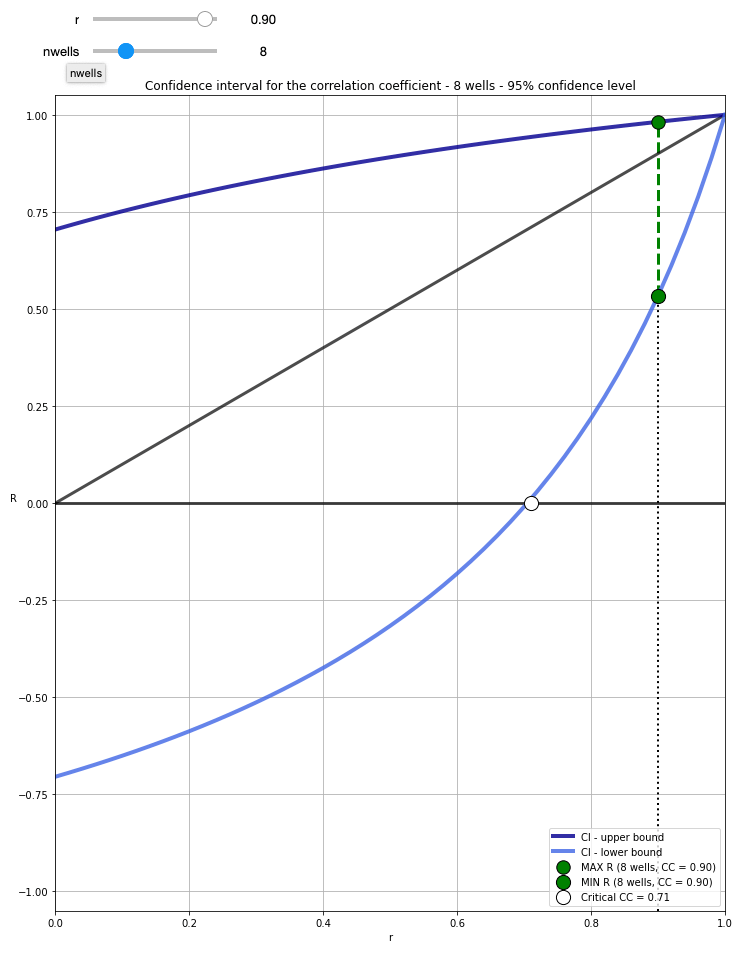With 9 wells, and cc=0.73, the resulting critical r = 0.67 tells us that for a 95% confidence level (0.05 alpha) we need at least a correlation coefficient of 0.67 in the sample (the 9 wells drilled) to be able to confidently say that there is correlation in the population (e.g. any well, future wells). However, the confidence interval is quite broad, ranging between 0.13 and 0.94 (you can get these numbers by running `confInt(0.73, 9)` in a cell.

With 8 wells (having removed the outlier), CC=0.9, the critical r is now 0.71, meaning that the requirement for rejecting the the null hypothesis (there is no association between Vp/Vs and Phi-H) is now a bit higher. However, a CC increased to 0.9, and only one less well, also results in a confidence interval ranging from 0.53 to 0.98, hence our confidence is greatly increased.

This second analysis also corroborates the choice of removing the outlier data point. One thing worth mentioning before moving on to the next test is that these confidence interval bounds are the expected population ones, based on the sample correlation coefficient and the number of observations; the data itself was not used. Of course, I could also have calculated, and shown you, the OLS regression confidence intervals, as I have done in this notebook (with a different dataset).

My final test involved using the distance correlation (`dcor.distance_correlation`) and p-value (`dcor.independence.distance_covariance_test`) from the `dcor` library. I have written before in a blog post how much I like the distance correlation, because it does not assume a linear relationship between variables, and because a distance correlation of zero does mean that there is no dependence between those two variables (contrary to Pearson and Spearman). In this case the relationship seems to be linear, but it is still a valuable test to compare DC and p-value before and after removing the outlier. Below is a summary of the test:
```All data points:
D.C. =  0.745
p-value =  0.03939
```
```Data without outlier:
D.C. =  0.917
p-value =  0.0012
```

The distance correlation values are very similar to the correlation coefficients from OLS, again going up once removed the outlier. But, more interestingly, with all data points, a p-value of 0.04079 is very close to the alpha of 0.05, whereas once we removed the outlier, the p-value goes down by a factor of 20, to 0.0018. This again backs up the decision to remove the outlier data point.